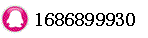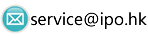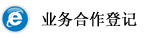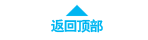# Volatility Arbitrage时间：2018-04-25 23:28:23     浏览：41    评论：0

Trading strategies that attempt to exploit differences between the forecasted future volatility of an asset and the implied volatility of options based on that asset. Because options pricing is determined by the volatility of the underlying asset, if the forecasted and implied volatilities differ, there will be a discrepancy between the expected price of the option and its actual market price.

A volatility arbitrage strategy is generally implemented through a delta neutral portfolio consisting of an option and its underlying asset. A long position in an option combined with a short position in the underlying asset is equivalent to a long volatility position. This strategy will be profitable if the realized volatility on the underlying asset eventually proves to be higher than the implied volatility on the option when the trade was initiated. Conversely, a short position in an option combined with a long position in the underlying asset is equivalent to a short volatility position, which will be profitable if the realized volatility on the underlying asset is ultimately lower than the option's implied volatility.

0相关评论联系我们